This tutorial shows different ways to add leading zeros in Excel: how to keep zeros as you type, show leading zeros in cells, remove or hide zeros.

If you use Excel not only to calculate numbers, but also maintain records such as zip codes, security numbers or employee ids, you may need to keep leading zeros in cells. However if you try typing a zip code like "00123" in a cell, Excel will immediately truncate it to "123".

The point is that Microsoft Excel treats postal codes, phone numbers and other similar entries as numbers, applies the General or Number format to them, and automatically removes preceding zeroes. Luckily, Excel also provides the means to keep leading zeros in cells, and further on in this tutorial you will find a handful of ways to do it.

## How to keep leading zeros in Excel as you type

For starters, let's see how you can put 0 in front of a number in Excel, for example type 01 in a cell. For this, simply change the cell format to Text:

• Select the cell(s) where you want to prefix numbers with 0.
• Go to the Home tab > Number group, and select Text in the Number Format box.

As soon as you type a zero(s) before number, Excel will display a small green triangle in the top-left corner of the cell indicating that something is wrong with the cell contents. To remove that error indicator, select the cell(s), click the warning sign, and then click Ignore Error.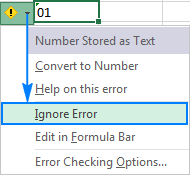The following screenshot shows the result: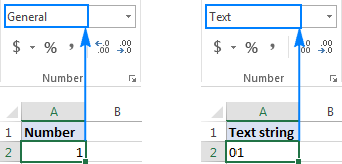Another way to keep leading zeros in Excel is to prefix a number with an apostrophe ('). For example, instead of typing 01, type '01. In this case, you don't need to change the cell's format.

Bottom line: This simple technique has a significant limitation - the resulting value is a text string, not number, and consequently it cannot be used in calculations and numeric formulas. If that is not what you want, then change only the visual representation of the value by applying a custom number format as demonstrated in the next example.

## How to show leading zeroes in Excel with custom number format

To display leading zeroes, apply a custom number format by performing these steps:

1. Select a cell(s) where you want to show leading zeros, and press Ctrl+1 to open the Format Cells dialog.
2. Under Category, select Custom.
3. Type a format code in the Type box.

In most cases, you will need a format code consisting of 0 placeholders, like 00. The number of zeros in the format code corresponds to the total number of digits you want to show in a cell (you will find a few examples below).

4. Click OK to save the changes.

For example, to add leading zeros to create a 5-digit number, use the following format code: 00000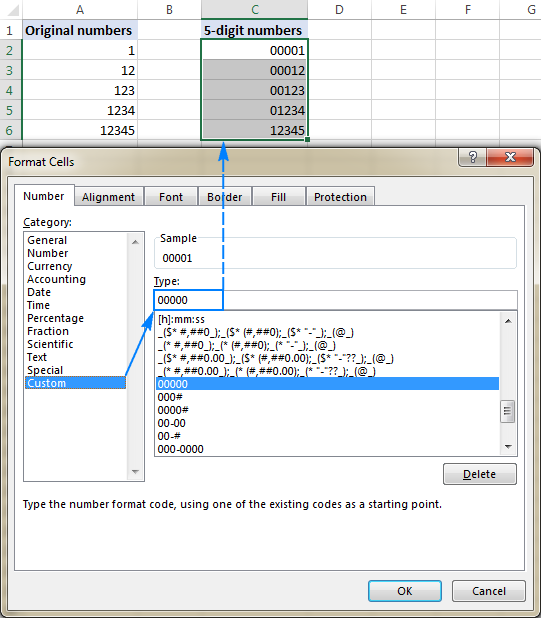By using Excel custom numbers formats, you can add leading zeros to create fixed-length numbers, like in the above example, and variable-length numbers. It all boils down to which placeholder you use in the format code:

• 0 - displays extra zeros
• # - does not display extra zeros

For example, if you apply the 000# format to some cell, any number you type in that cell will have up to 3 leading zeros.

Your custom number formats can also include spaces, hyphens, parentheses, etc. The detailed explanation can be found here: Custom Excel number format.

The following spreadsheet gives a few more examples of custom formats that can show leading zeros in Excel.

A B C
1 Custom format Typed number Displayed number
2 00000 123 00123
3 000# 123 0123
4 00-00 1 00-01
5 00-# 1 00-1
6 000-0000 123456 012-3456
7 ###-#### 123456 12-3456

And the following format codes can be used to display numbers in special formats such us zip codes, phone numbers, credit card numbers, and social security numbers.

A B C D
1   Custom format Typed number Displayed number
2 Zip code 00000 1234 01234
3 Social security 000-00-0000 12345678 012-34-5678
4 Credit card 0000-0000-0000-0000 12345556789123 0012-3455-5678-9123
5 Phone numbers 00-0-000-000-0000 12345556789 00-1-234-555-6789

Tip. Excel has a few predefined Special formats for postal codes, telephone numbers and social security numbers, as shown in the screenshot below: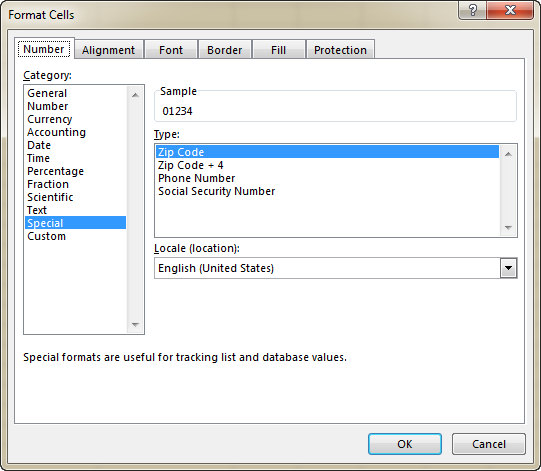Bottom line: This method is best to be used in situations when you work with a numeric dataset and the results should be numbers, not text. It changes only the display of a number, but not the number itself: leading zeros show up in cells, the actual value displays in the formula bar. When you reference such cells in formulas, the calculations are perfumed with the original values. Custom formats can only be applied to numeric data (numbers and dates) and the result is also a number or date.

## How to add leading zeros in Excel with the TEXT function

While a custom number format shows zero in front of a number without actually changing the underlying value, the Excel TEXT function pads numbers with zeros by "physically" inserting leading zeros in cells.

To add leading zeros with a TEXT(value, format_text) formula, you use the same format codes as in custom number formats. However, the result of the TEXT function is always a text string, even if it looks much like a number.

For example, to insert 0 before a value in cell A2, use this formula:

`=TEXT(A2, "0#")`

To create a zero-prefixed string of a fixed length, say a 5-character string, use this one:

`=TEXT(A2, "000000")`

Please pay attention that the TEXT function requires enclosing the format codes in quotation marks. And this is how the results will look like in Excel:

A B C
1 Original number Padded number Formula
2 1 01 =TEXT(B2, "0#")
3 12 12 =TEXT(B3, "0#")
4 1 00001 =TEXT(B4,"00000")
5 12 00012 =TEXT(B5,"00000")

Bottom line: The Excel TEXT function always returns a text string, not number, and therefore you won't be able to use the results in arithmetic calculations and other formulas, unless you need to compare the output with other text strings.

In the previous examples, you learned how to add zero before a number in Excel. But what if you need to put zero(s) in front of a text string like 0A102? In that case, neither TEXT nor custom format will work because they deal with numeric values only.

If the value to be padded with zero contains letters or other text characters, use one of the following formulas, which offer a universal solution applicable to both numbers and text strings.

The easiest way to put leading zeros before text strings in Excel is using the RIGHT function:

RIGHT("0000" & cell, string_length)

Where:

• "0000" is the maximum number of zeros you want to add. For example, to add 2 zeros, you type "00".
• Cell is a reference to the cell containing the original value.
• String_length is how many characters the resulting string should contain.

For example, to make a zero-prefixed 6-character string based on a value in cell A2, use this formula:

`=RIGHT("000000"&A2, 6)`

What the formula does is add 6 zeros to the value in A2 ("000000"&A2), and then extract the right 6 characters. As the result, it inserts just the right number of zeros to reach the specified total string limit: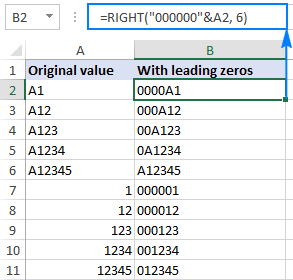In the above example, the maximum number of zeros equals the total string length (6 characters), and therefore all of the resulting strings are 6-character long (fixed length). If applied to a blank cell, the formula would return a string consisting of 6 zeros.

Depending on your business logic, you can supply different numbers of zeros and total characters, for example:

`=RIGHT("00"&A2, 6)`

As the result, you will get variable-length strings that contain up to 2 leading zeros: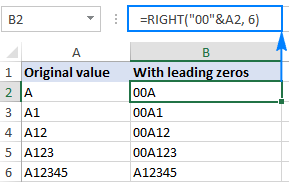### Formula 2. Pad leading zeros using the REPT and LEN functions

Another way to insert leading zeros before a text string in Excel is using this combination of REPT and LEN functions:

REPT(0, number of zeros-LEN(cell))&cell

For example, to add leading zeroes to the value in A2 to create a 6-character string, this formula goes as follows:

`=REPT(0, 6-LEN(A2))&A2`

#### How this formula works:

Knowing that the REPT function repeats a given character a specified number of times, and LEN returns the total length of the string, the formula's logic is easy to understand:

• LEN(A2) gets the total number of characters in cell A2.
• REPT(0, 6-LEN(A2)) adds the required number of zeros. To calculate how many zeros should be added, you subtract the length of the string in A2 from the maximum number of zeros.
• Finally, you concatenate zeros with the A2 value, and get the following result: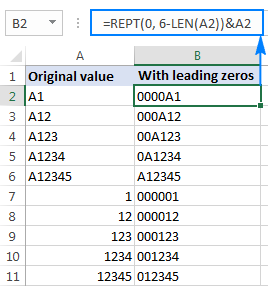Bottom line: This formula can add leading zeros both to numbers and text strings, but the result is always text, not number.

## How to add a fixed number of preceding zeros

To prefix all values in a column (numbers or text strings) with a certain number of zeros, use the CONCATENATE function, or the CONCAT function in Excel 365 - 2019, or the ampersand operator.

For example, to put 0 before a number in cell A2, use one of these formulas:

`=CONCATENATE(0,A2)`

or

`=0&A2`

As shown in the screenshot below, the formula adds just one leading zero to all cells in a column regardless of how many characters the original value contains: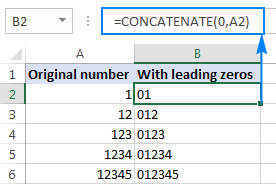In the same manner, you can insert 2 leading zeros (00), 3 zeros (000) or as many zeros as you want before numbers and text strings.

Bottom line: The result of this formula is also a text string even when you are concatenating zeros with numbers.

## How to remove leading zeros in Excel

The method you use to remove leading zeros in Excel depends on how those zeros were added:

• If preceding zeroes were added with a custom number format (zeros are visible in a cell, but not in the formula bar), apply another custom format or revert back General as shown here.
• If zeros were typed or otherwise entered in cells formatted as Text (a small green triangle is displayed in the top-left corner of the cell), convert text to number.
• If leading zeroes were added by using a formula (the formula appears in the formula bar when the cell is selected), use the VALUE function to remove them.

The following image shows all three cases to help you choose the right technique: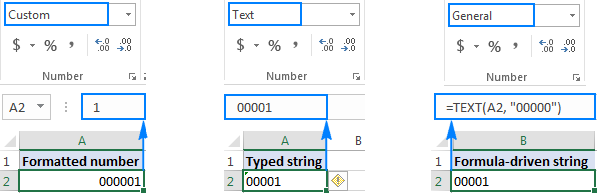### Remove leading zeros by changing the cell format

If leading zeroes are shown in cells with a custom format, then change the cell format back to default General, or apply another number format that does not display preceding zeros.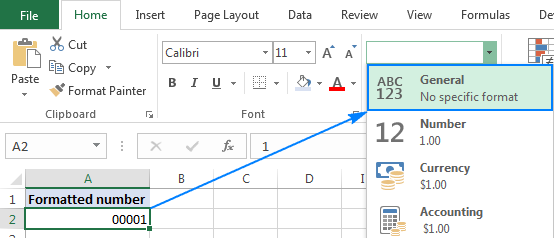### Remove leading zeros by converting text to number

When prefixed zeros appear in a Text-formatted cell, the easiest way to remove them is select the cell(s), click the exclamation point, and then click Convert to Number: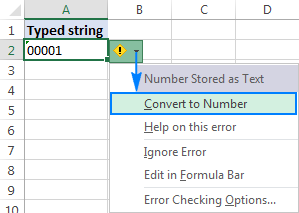### Remove leading zeros by using a formula

In case a preceding zero(s) is added with a formula, use another formula to remove it. The zero-removing formula is as simple as:

`=VALUE(A2)`

Where A2 is the cell from which you want to remove preceding zeros.

This method can also be used to get rid of zeros typed directly in cells (like in the previous example) or imported to Excel from some external source. Overall, if you are dealing with a zero-prefixed string that represents a number, you can use the VALUE function to convert text to number and remove leading zeros along the way.

The following screenshot shows two formulas:

• The Text formula in B2 adds zeros to the value in A2, and
• The Value formula in C2 removes the leading zeros from the value in B2.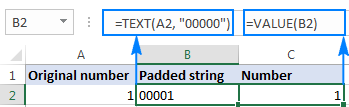## How to hide zeros in Excel

If you don't want to display zero values in your Excel sheet, you have the following two options:

1. To hide zeros across the entire sheet, uncheck the Show a zero in cells that have zero value option. For this, click File > Options > Advanced, and scroll down to the Display options for this worksheet section: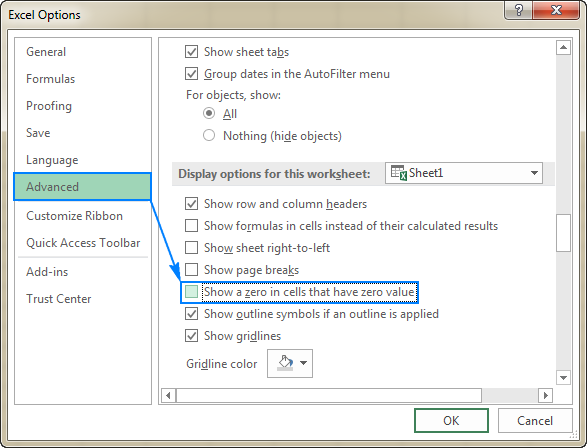2. To hide zero values in certain cells, apply the following custom number format to those cells: #;#;;@

For this, select the cells where you want to hide zeros, click Ctrl+1 to open the Format Cells dialog, select Custom under Category, and type the above format code in the Type box.

The screenshot below shows that cell B2 does contain a zero value, but it is not displayed in the cell: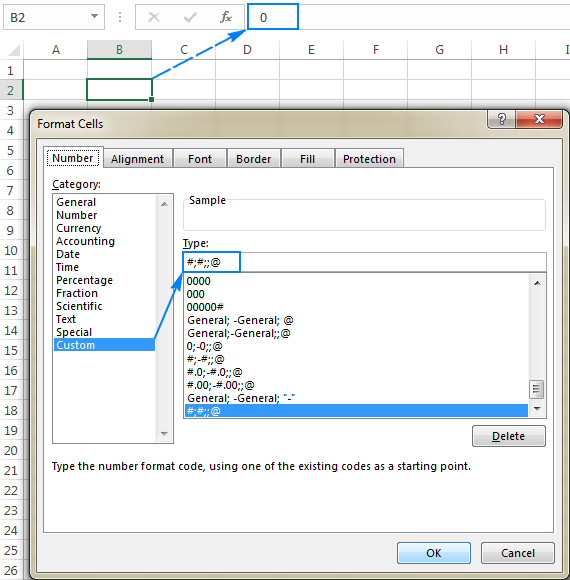## Add and remove zeros in Excel an easy way

Finally, good news for users of our Ultimate Suite for Excel - a new tool especially designed for handling zeros is released! Please welcome Add/Remove Leading Zeros.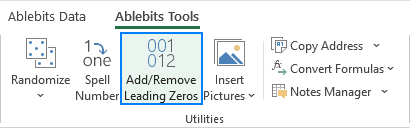As usual, we've strived to reduce the number of moves to an absolute minimum :)

1. Select the target cells and run the Add/Remove Leading Zeros tool.
2. Specify the total number of characters that should be displayed.
3. Click Apply.

Done!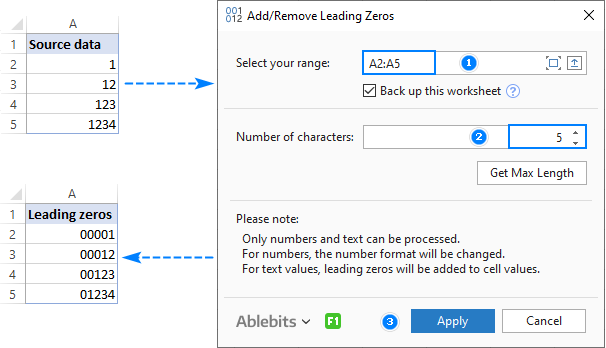To remove leading zeros, the steps are very much alike:

2. Specify how many characters should be displayed. To get the maximum number of significant digits in the selected range, click the Get Max Length
3. Click Apply.

• For numbers, a custom number format is set, i.e. only a visual representation of a number is changed, not the underlying value.
• Alpha-numeric strings are prefixed with leading zeros, i.e. zeroes are physically inserted in cells.

This is how you can add, remove and hide zeros in Excel. To better understand the techniques described in this tutorial, you are welcome to download the sample workbook. I thank you for reading and hope to see you on our blog next week!

Excel Leading Zeros examples (.xlsx file)
Ultimate Suite 14-day fully-functional version (.exe file)

## You may also be interested in

1. For 12345_1, display 12345_001

• Hi! Insert the characters "00" into the text as described in these instructions: How to add text or character to every cell in Excel. Try this formula:

=LEFT(A2, SEARCH("_", A2)) &"00"& RIGHT(A2, LEN(A2) - SEARCH("_", A2))

Or you can extract the number after the "_" character with the MID function and convert it to text in the desired format with the TEXT function. For example:

=LEFT(A2,SEARCH("_",A2))&TEXT(--MID(A2,SEARCH("_",A2)+1,10),"000")

How to do this in Excel- "00 ASDF 123 TO 00123 ASDF"

• I don't want split the text. I want to rearrange word place in one cell.

For Example: EMPLOYEE FILE ; EMPLOYEE MARTIN 123

I want like this: EMPLOYEE FILE ; EMPLOYEE 123 MARTIN

• To rearrange words, you can at first split text into separate words.

3. How to :

for 1:2 Display 001:02
for 13:8 Display 013:08

• Hi! These are not numbers, but text. Therefore, you cannot automatically add leading zeros using format cell.

4. Saya punya data
C1b2
A1
A1b2c3

C01b02
A01
A01b02c03

Apakah ada formula langsungnya? Terima kasih

• I'm really sorry, looks like this is not possible with the standard Excel options.

5. Hi Sir, I want to remove only one leading zero from this number: 0004879658954.
How can i do it sir?
I appreciate your kind consideration. Thanks!

• Formula worked.
Thanks a lot, Sir.

6. Hi,
is it possible to add zeros between letters and numbers?
From:
LK1
LK3
LK10
LK12
LK100

I would like to get:
LK001
LK003
LK010
LK012
LK100

Thanks!

• Hello!
You can extract characters from a text string starting from a certain position with the MID function. Then use the recommendations from the article above.

=LEFT(A1,2)&TEXT(--MID(A1,3,5),"000")

7. Once the original data has come into my excel CSV file, and excel has already dropped the leading zero(s), how can I restore the leading zeros back?

For example, if 00329384 comes in but excel displays 329384
For example, if 0329384 comes in but excel displays 329384

Even when I change the format to "text", the zero's are still gone. Is the original data with the leading zero's still contained in the excel file somewhere?

• Hello!
To preserve leading zeros, treat data as text. You cannot recover leading zeros in numbers.

• This page helped me solve my work problem. Thanks so much.

8. Excelente information

Regards from Honduras CA-

9. Hi,
I'm trying to create a formula that will turn a cell such as "3.5.12.5 Introduction" into "03.05.12.05" for a Table of Contents solution. The formula I have functions properly before the first decimal, but I'm having trouble altering it to apply to all.

=IF(ISNUMBER(VALUE(LEFT(B5,1))),IF(VALUE(LEFT(B5,FIND(".",B5)-1))>9,LEFT(B5,FIND(" ",B5)-1),"0"&LEFT(B5,FIND(" ",B5)-1)))

Any help would be appreciated!
The solution below works in excel online but I need it to function in excel desktop/SharePoint-
=TEXTJOIN(".",TRUE,IF(ISNUMBER(TEXTSPLIT(B5,{".";" "})+0),TEXT(TEXTSPLIT(B5,{".";" "}),"00"),""))

• Hi!
The result of the formula is "03.05.12.05". If this is not what you need, explain in detail what result you want to get.

• Hi,
I'm hoping to use a formula that doesn't use TEXTSPLIT.
Thanks!

10. How can I define 1 decimal at most (ıf the number does not have any decimals, display should be a whole number). "#.#" does not give the format I want since if there is no decimals then it displays a dot after the whole number.

For "125.2" display "125.5"
For "125,24" display "125.2"
For "125" display "125" (not "125.")

• Hello!
To avoid showing the decimal separator in integers, use conditional formatting. Conditional formatting formula:

=A1=INT(A1)

Apply number format when condition is met: #,###

11. I wonder if anyone can help me. I am doing a project and I come across a brick wall. What I would like to do on my spreadsheet is to add a blank row above using a button (VBA) then I would want the cells in column A to automatically have a serial number in this format... ABCD001. When I click a button it will add the row above this and it will generate a value of ABCD002.

12. It was easy to locate what I wanted to do. The instructions were clear and the example formula showed exactly what to do. Thank you!

Do you have any suggestions on adding 0's before dates?

I usually receive them in the text format, so I split it up by the forward slash (/), add in the 0's manually by carefully sorting each column, and then concatenate them back together. Is there a better way?

Also, is there a way to convert the dates into text without them turning into their raw values?

• Hello!
With the & operator, you can add a zero before the text date.

• Thank you for your help! I am now using `=TEXT(CELL,"MM/DD/YYYY")` and it works like a charm.

14. I have a table
A B C
01 01 01

If i concatenate it 111 is coming. But i need 010101. Can you tell me the process?

• Hi!
If "01" is written in the cell, then this is text. Use the & operator or the CONCATENATE function. You can write "01" in a cell with an apostrophe. Re-check also the paragraph above: How to add leading zeros in Excel with the TEXT function

15. ALIAS NAME
72019195
2093573
72010822
72014127
1191408
72010821
1191407
10021026
10021007
10021000
72016400
72016401
10019242
72013368
2030021
2030285
2030042
2030151
2030164
2030142
72000538
2060217
72016399
2060129
2060112
4033444
2060061
2060164
1110318
1191437
1150012

I want to add ''0" in the front for those 7 digit number. Not all

Any Formula pls